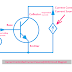## Definition/Concept

The current-controlled current source is where the output current or source current to the output circuit depends upon the current flow elsewhere in the circuit. So the controlling current elsewhere in the circuit can control the output current. In short, it is known as CCCS. It is a type of dependent source. I may sure it is a little bit confusing to understand this topic for you. Don't worry, read the whole article, your doubt will be clear.

We know that there are four types of dependent sources VCVS, CCVS, VCCS, and CCCS. In the previous articles, we have discussed all three of them. Now we are going to understand CCCS.

So, when we use a current source for any applications and the amount of current supplied by that source is dependent or controlled by the current flow elsewhere in that current source circuit itself then it is considered as a Controlled current source. We can say the controlling current determined the magnitude of the output current.

The current controlled current output is determined by the equation, IOUT = βIIN

Here,  IOUT = Output Current
IIN  = Input Current
β(beta) = magnifying constant, sometimes it is known as current transfer ratio

Remember that there is no unit of this current transfer ratio β(beta) because, β = IOUT/IIN

so you may understand there will be no unit for ampere/ampere.

## Current Controlled Current Source(CCCS) Circuit Diagram

Here, you can see a very simple and basic circuit diagram of CCCS to understand its concept. We have taken a bipolar junction transistor. where the base current is the controlling current and the emitter current is the dependent current.

We know that the bipolar junction transistor is a current-controlled device. When we vary the base current the current flow through the emitter and collector also changes or varies.

## CCCS Symbol

Here, you can see the symbol of the Current Controlled Current Source. The left section shows the controlling current and the right section shows the controlled or dependent current.

## CCCS Examples

As we already see in the above circuit diagram, the practical example of CCCS is Bipolar Junction Transistor(BJT) where the emitter current is controlled by the base current.

What is CCCS(Current Controlled Current Source)? ExamplesReviewed by Author on 6/18/2022 Rating: 5